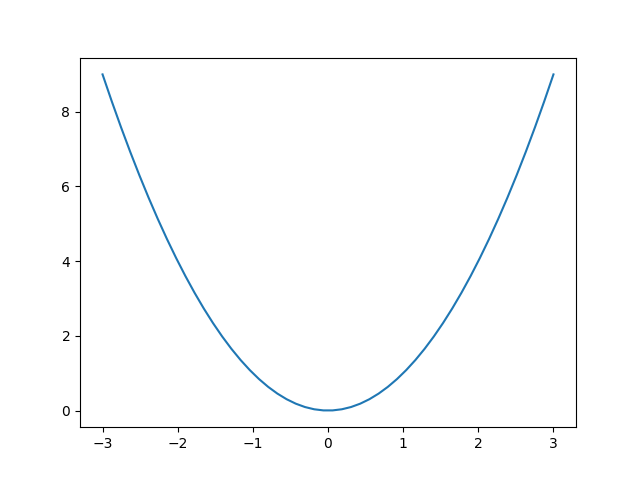Travis-CI:# Timers¶

Simple example of using general timer objects. This is used to update the time placed in the title of the figure.```import matplotlib.pyplot as plt
import numpy as np
from datetime import datetime

def update_title(axes):
axes.set_title(datetime.now())
axes.figure.canvas.draw()

fig, ax = plt.subplots()

x = np.linspace(-3, 3)
ax.plot(x, x ** 2)

# Create a new timer object. Set the interval to 100 milliseconds
# (1000 is default) and tell the timer what function should be called.
timer = fig.canvas.new_timer(interval=100)
timer.start()

# Or could start the timer on first figure draw
#def start_timer(evt):
#    timer.start()
#    fig.canvas.mpl_disconnect(drawid)
#drawid = fig.canvas.mpl_connect('draw_event', start_timer)

plt.show()
```

Total running time of the script: ( 0 minutes 0.033 seconds)

Gallery generated by Sphinx-Gallery